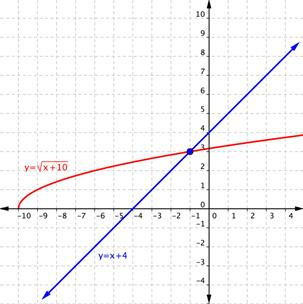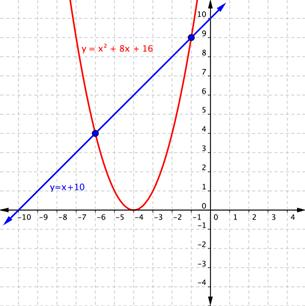### Learning Outcomes

• Isolate a radical term in an equation using algebra and rules for radicals and exponents
• Identify a radical equation with no solution or extraneous solutions

An equation that contains a radical expression is called a radical equation. Solving radical equations requires applying the rules of exponents and following some basic algebraic principles. In some cases, it also requires looking out for errors generated by raising unknown quantities to an even power.

A basic strategy for solving radical equations is to isolate the radical term first, and then raise both sides of the equation to a power to remove the radical. The reason for using powers will become clear in a moment. This is the same type of strategy you used to solve other, non-radical equations—rearrange the expression to isolate the variable you want to know, and then solve the resulting equation.

There are two key ideas that you will be using to solve radical equations. The first is that if $a=b$, then ${{a}^{2}}={{b}^{2}}$. This property allows you to square both sides of an equation and remain certain that the two sides are still equal. The second is that if the square root of any nonnegative number x is squared, then you get x: ${{\left( \sqrt{x} \right)}^{2}}=x$. This property allows you to “remove” the radicals from your equations.

Let us start with a radical equation that you can solve in a few steps:$\sqrt{x}-3=5$.

### Example

Solve. $\sqrt{x}-3=5$

To check your solution, you can substitute $64$ in for x in the original equation. Does $\sqrt{64}-3=5$? Yes—the square root of $64$ is $8$, and $8−3=5$.

Notice how you combined like terms and then squared both sides of the equation in this problem. This is a standard method for removing a radical from an equation. It is important to isolate a radical on one side of the equation and simplify as much as possible before squaring. The fewer terms there are before squaring, the fewer additional terms will be generated by the process of squaring.

In the example above, only the variable x was underneath the radical. Sometimes you will need to solve an equation that contains multiple terms underneath a radical. Follow the same steps to solve these, but pay attention to a critical point—square both sides of an equation, not individual terms. Watch how the next two problems are solved.

### Example

Solve. $\sqrt{x+8}=3$

In the following video example, you will see two more examples that are similar to the ones above.

### Example

Solve. $1+\sqrt{2x+3}=6$

2. Square both sides of the equation: If $x=y$, then $x^{2}=y^{2}$.
3. Once the radical is removed, solve for the unknown.

## Identify a Radical Equation with No Solutions or Extraneous Solutions

Following rules is important, but so is paying attention to the math in front of you—especially when solving radical equations. Take a look at this next problem that demonstrates a potential pitfall of squaring both sides to remove the radical.

### Example

Solve. $\sqrt{a-5}=-2$

Look at that—the answer $a=9$ does not produce a true statement when substituted back into the original equation. What happened?

Check the original problem: $\sqrt{a-5}=-2$. Notice that the radical is set equal to $−2$, and recall that the principal square root of a number can only be positive. This means that no value for a will result in a radical expression whose positive square root is $−2$! You might have noticed that right away and concluded that there were no solutions for a. But why did the process of squaring create an answer, $a=9$, that proved to be incorrect?

The answer lies in the process of squaring itself. When you raise a number to an even power—whether it is the second, fourth, or $50$th power—you can introduce a false solution because the result of an even power is always a positive number. Think about it: $3^{2}$ and $\left(−3\right)^{2}$ are both $9$, and $2^{4}$ and $\left(−2\right)^{4}$ are both $16$. So when you squared $−2$ and got $4$ in this problem, you artificially turned the quantity positive. This is why you were still able to find a value for a—you solved the problem as if you were solving$\sqrt{a-5}=2$! (The correct solution to $\sqrt{a-5}=-2$ is actually “no solution.”)

Incorrect values of the variable, such as those that are introduced as a result of the squaring process are called extraneous solutions. Extraneous solutions may look like the real solution, but you can identify them because they will not create a true statement when substituted back into the original equation. This is one of the reasons why checking your work is so important—if you do not check your answers by substituting them back into the original equation, you may be introducing extraneous solutions into the problem.

Have a look at the following problem. Notice how the original problem is $x+4=\sqrt{x+10}$, but after both sides are squared, it becomes ${{x}^{2}}+8x+16=x+10$. Squaring both sides may have introduced an extraneous solution.

In the following video, we present more examples of solving radical equations by isolating a radical term on one side.

### Example

Solve. $x+4=\sqrt{x+10}$

It may be difficult to understand why extraneous solutions exist at all. Thinking about extraneous solutions by graphing the equation may help you make sense of what is going on.

You can graph $x+4=\sqrt{x+10}$ on a coordinate plane by breaking it into a system of two equations: $y=x+4$ and $y=\sqrt{x+10}$. The graph is shown below. Notice how the two graphs intersect at one point, when the value of x is $−1$. This is the value of x that satisfies both equations, so it is the solution to the system.Now, following the work we did in the example problem, let us square both of the expressions to remove the variable from the radical. Instead of solving the equation $x+4=\sqrt{x+10}$, we are now solving the equation $\left(x+4\right)^{2}=\left(\sqrt{x+10}\right)^{2}$ , or $x^{2}+8x+16=x+10$. The graphs of $y=x^{2}+8x+16$ and $y=x+10$ are plotted below. Notice how the two graphs intersect at two points, when the values of x are $−1$ and $−6$.Although $x=−1$ is shown as a solution in both graphs, squaring both sides of the equation had the effect of adding an extraneous solution, $x=−6$. Again, this is why it is so important to check your answers when solving radical equations!

### Example

Solve. $4+\sqrt{x+2}=x$

In the next video, we show another example of radical equations that have extraneous solutions. Always remember to check your answers for radical equations.

In our last example, we will use a difference of squares to solve a radical equation with two radicals. You will see that we have to isolate and eliminate one radical first, and then tackle isolating and eliminating the other. We will also use factoring techniques for trinomials and the zero product principle to solve this equation.

### Example

Solve $\sqrt{2x+3}+\sqrt{x - 2}=4$.

In our last video, we show the solution for another radical equation that has square roots on both sides of the equation. Pay special attention to how you square the side that has the sum of a radical term and a constant.

Radical equations play a significant role in science, engineering, and even music. Sometimes you may need to use what you know about radical equations to solve for different variables in these types of problems.

### Summary

A common method for solving radical equations is to raise both sides of an equation to whatever power will eliminate the radical sign from the equation. But be careful—when both sides of an equation are raised to an even power, the possibility exists that extraneous solutions will be introduced. When solving a radical equation, it is important to always check your answer by substituting the value back into the original equation. If you get a true statement, then that value is a solution; if you get a false statement, then that value is not a solution.